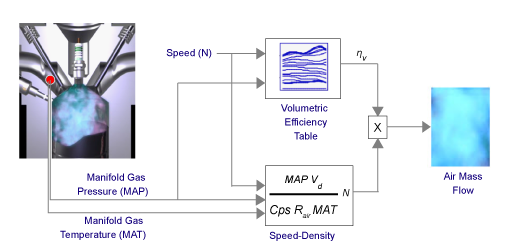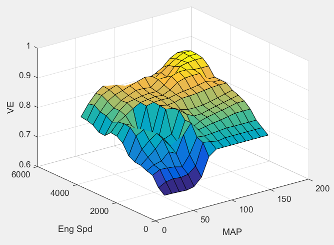## SI Engine Speed-Density Air Mass Flow Model

To calculate the air mass flow in the spark-ignition (SI) engine, you can configure the Spark Ignition Core Engine block to use a speed-density air mass flow model. The speed-density model uses the speed-density equation to calculate the engine air mass flow. The equation relates the engine air mass flow to the intake manifold gas pressure, intake manifold gas temperature, and engine speed. Consider using this air mass flow model in simple conventional engine designs, where variable valvetrain technologies are not in use.To determine the air mass flow, the speed-density air mass flow model applies these speed-density equations at the intake manifold gas pressure and gas temperature states.

The speed-density air mass flow model uses a volumetric efficiency lookup table to correct the ideal air mass flow.

The engine volumetric efficiency lookup table, ${f}_{{\eta }_{v}}$, is a function of intake manifold absolute pressure and engine speed

`${\eta }_{v}={f}_{{\eta }_{v}}\left(MAP,N\right)$`

where:

• ${\eta }_{v}$ is engine volumetric efficiency, dimensionless.

• MAP is intake manifold absolute pressure, in KPa.

• N is engine speed, in rpm.To develop the volumetric efficiency table, use the measured air mass flow rate, intake manifold gas pressure, intake manifold gas temperature, and engine speed from engine performance testing.

The air mass flow model implements equations that use these variables.

 $MAP$ Cycle average intake manifold pressure ${\stackrel{˙}{m}}_{intk}$ Engine intake port mass flow ${\stackrel{˙}{m}}_{air}$ Engine intake air mass flow ${V}_{d}$ Displaced volume N Engine speed $Cps$ Crankshaft revolutions per power stroke $MAT$ Cycle average intake manifold gas absolute temperature ${R}_{air}$ Ideal gas constant for air and burned gas mixture ${f}_{{\eta }_{v}}$ Engine volumetric efficiency lookup table ${\eta }_{v}$ Engine volumetric efficiency

 Heywood, John B. Internal Combustion Engine Fundamentals. New York: McGraw-Hill, 1988.